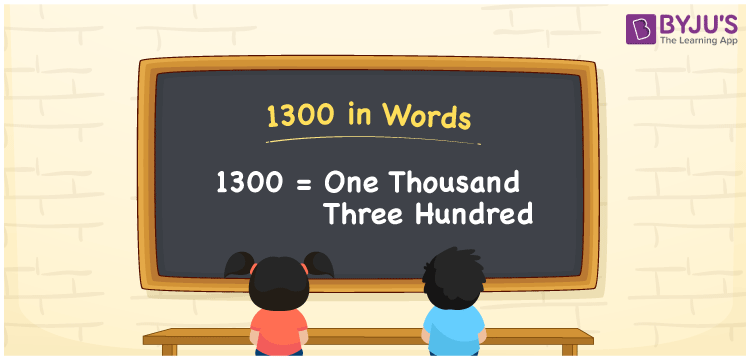# 1300 in Words

1300 in words is written as One Thousand Three Hundred. The number name of 1300 is derived using the ones, tens, hundreds, thousands place of the number. Therefore, a place value chart is very important in writing the number 1300 in words. For example, if you have purchased a handbag worth Rs 1300, then you can write, “I have purchased a handbag worth Rs One Thousand Three Hundred”. 1300 is a cardinal number as it shows a specific quantity.

 1300 in words One Thousand Three Hundred One Thousand Three Hundred in numerical form 1300

## 1300 in English Words

Here, we learn to write the numbers in words using English alphabets. Hence, 1300 in English is written as ‘One Thousand Three Hundred’.## How to Write 1300 in Words?

The place value chart is essential to convert any number to words. The number 1300 has 4 digits. The below table shows the place value chart for the number 1300.

 Thousands Hundreds Tens Ones 1 3 0 0

Thus, we can write the expanded form as:

1 x Thousand + 3 x Hundred + 0 x Tens + 0 x Ones

= 1 x 1000 + 3 x 100 + 0 x 10 + 0 x 1

= 1000 + 300

= 1300

= One Thousand Three Hundred

Therefore, 1300 in words is written as One Thousand Three Hundred.

Interesting way of writing 1300 in words

1 = One

13 = Thirteen

130 = One Hundred and Thirty

1300 = One Thousand Three Hundred

1300 is a natural number that is succeeded by 1299 and preceded by 1301

• 1300 in words – One Thousand Three Hundred
• Is 1300 an odd number? – No
• Is 1300 an even number? – Yes
• Is 1300 a perfect square number? – No
• Is 1300 a perfect cube number? – No
• Is 1300 a prime number? – No
• Is 1300 a composite number? – Yes

## Frequently Asked Questions on 1300 in Words

Q1

### Write the spelling of 1300.

The spelling of 1300 is One Thousand Three Hundred.
Q2

### Find the value 2000 – 700 and write the answer in words.

On simplification of 2000 – 700, we get 1300. Hence, 1300 in words is One Thousand Three Hundred.
Q3

### Is the number 1300 an even number?

Yes, the number 1300 is an even number.
Test your Knowledge on 1300 in Words